Mathematical and Physical Journal
for High Schools
Issued by the MATFUND Foundation
 Already signed up? New to KöMaL?

# KöMaL Problems in Physics, October 2018

Show/hide problems of signs:## Problems with sign 'M'

Deadline expired on November 12, 2018.

M. 380. Measure the rotational inertia about the symmetry axis of a hard boiled egg.

(6 pont)

statistics## Problems with sign 'G'

Deadline expired on November 12, 2018.

G. 645. In a NASA' vacuum chamber it was filmed that both a hammer and a feather fall towards the Earth at the same acceleration of $\displaystyle g=9.81~\rm m/s^2$, and if they are released at the same instant then they hit the ground at the same time. What will the acceleration of the feather and the hammer be if the film is played at twice the speed of the recording?

(3 pont)

solution (in Hungarian), statistics

G. 646. In a chemistry laboratory chemicals are stored in alike bottles. One is full of glycerine, and the other is full of ether. The mass of the bottle filled with glycerine is 2290 grams, and that of the other bottle with ether in it has a mass of 1471 grams. What is the mass of an empty bottle?

(3 pont)

solution (in Hungarian), statistics

G. 647. In two – seemingly alike – electric kettles the heating wire is bent into the shape of a regular hexagon. In one of the kettles the heating element was connected as shown in figure $\displaystyle a)$, whilst in the other the heating element was connected as shown in figure $\displaystyle b)$. In which kettle will the water start to boil sooner?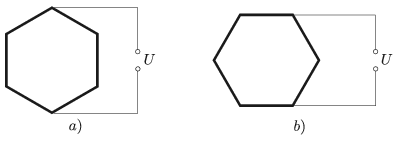(3 pont)

solution (in Hungarian), statistics

G. 648. A small bug starts crawling from the vertex $\displaystyle P$ of a wooden cube of edges 10 cm. At least how long does it take for the bug to reach the furthest vertex $\displaystyle Q$ of the cube, if the speed of the bug is 1 cm/s? In how many different paths can the bug move in order to reach $\displaystyle Q$ in the shortest time?

(4 pont)

solution (in Hungarian), statistics## Problems with sign 'P'

Deadline expired on November 12, 2018.

P. 5056. A spring with negligibly small mass and of spring constant 40 N/m is standing on the tabletop in a vertical position, and on its top there is a sheet, which also has negligible mass. A 0.2 kg small object is dropped to the sheet from a height of 0.4 m, measured from the level of the sheet. For how long will the small object be on the sheet if it does not stick to it?

(5 pont)

solution (in Hungarian), statistics

P. 5057. A small object of mass $\displaystyle m=0.5$ kg and another of mass $\displaystyle 3m$ are placed to an inclined plane of angle of elevation of $\displaystyle \alpha=30^\circ$. The two small objects are attached with a negligible-mass rigid rod of length $\displaystyle d=50$ cm. The top part of the slope is frictionless, whilst on the bottom part of the slope the coefficient of friction is $\displaystyle \mu=0.2$.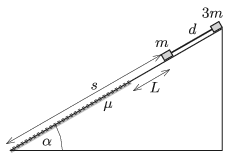Initially the object of mass $\displaystyle m$ was at a distance of $\displaystyle L=40$ cm from the boundary of that region where there is friction, and at a distance of $\displaystyle s=120$ cm from the bottom of the plane. The system of the two (point-like) objects is released.

$\displaystyle a)$ Give the force exerted in the rod as a function of the distance covered.

$\displaystyle b)$ How long does it take for the object of mass $\displaystyle m$ to reach the bottom of the inclined plane?

(5 pont)

solution (in Hungarian), statistics

P. 5058. A whimsical Alaskan constructor runs a strange adventure park. Inside a very tall iceberg he builds a helical bob sleigh track. The symmetry axis of the helical path is vertical, its diameter is $\displaystyle d$, and the lead of the path is $\displaystyle h$. The track starts at the top of the iceberg and at the bottom of the iceberg it ends after a short frictionless bend, in a horizontal, straight path of length $\displaystyle s$. The path is very long (for the passengers it seems infinitely long''), the bobs have neither steering wheel nor brake in them, and they stop just at the end of the horizontal part of the track. (For the sake of simplicity consider the bob sleighs point-like objects.)

$\displaystyle a)$ What is the coefficient of kinetic friction between the metal body of the bob and the ice?

$\displaystyle b)$ What is the greatest speed of the bobs?

Data: $\displaystyle d=10$ m, $\displaystyle h=1.5$ m, $\displaystyle s=270$ m.

(5 pont)

solution (in Hungarian), statistics

P. 5059. How long does an object take to fall into the Sun from a distance of 50 AU from the Sun, if it starts without initial speed? How long does it take to cover half of the distance?

(5 pont)

solution (in Hungarian), statistics

P. 5060. Two alike glass balloons are connected with a thin, short tube, whose inner volume is negligibly small. The temperature of the air inside the balloons is $\displaystyle 27~{}^\circ$C. By what percent does the pressure of the enclosed air in the balloons increase, if one of the balloons is heated to a temperature of $\displaystyle 177~{}^\circ$C, whilst the other is kept at the temperature of $\displaystyle 27~{}^\circ$C?

(4 pont)

solution (in Hungarian), statistics

P. 5061. The equation of the process through which a sample of ideal gas of constant mass taken is the following: $\displaystyle pV^n=\text{constant}$. $\displaystyle a)$ What is the value of $\displaystyle n$ if the process is isothermal, isobaric or adiabatic? $\displaystyle b)$ What can the value of $\displaystyle n$ be in the case of air, if the gas releases heat during the process, and still heats up?

(4 pont)

solution (in Hungarian), statistics

P. 5062. From experimental results we know that the resistance of conductors depends on their temperature. For some alloys this temperature coefficient of resistance is negative, whilst for some others it is positive. Hence, by connecting pieces of wires of different alloys, one can create a wire whose resistance is independent of the temperature in a wide temperature range. In the table below, for two alloys manganin and constantan, the resistance values $\displaystyle r$ of unit-length wires measured at $\displaystyle 0~{}^\circ$C, and their temperature coefficient of resistance $\displaystyle \alpha$ are given.

 $\displaystyle r\;[\Omega/\rm m]$ $\displaystyle \alpha\;[1/{}^\circ\rm C]$ constantan 6.3 $\displaystyle -5{.}0\cdot 10^{-5}$ manganin 5.3 $\displaystyle +1{.}4\cdot 10^{-5}$

What length of wires made from manganin and constantan should be connected in series in order to gain an equivalent resistance of $\displaystyle 5{.}0~\Omega$ which is independent of the temperature?

(3 pont)

solution (in Hungarian), statistics

P. 5063. The meters in the circuit shown in the figure are ideal and their readings are indicated. Find the resistance values of the $\displaystyle R_1$, $\displaystyle R_2$, $\displaystyle R_3$ and $\displaystyle R_4$ resistors.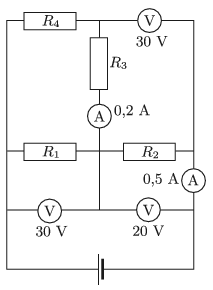(4 pont)

solution (in Hungarian), statistics

P. 5064. A copper ring of mass $\displaystyle m_2=0{.}05$ kg and of charge $\displaystyle Q=8\cdot10^{-6}$ C is attached to the rim of an insulating disc of mass $\displaystyle m_1=0{.}2$ kg and of radius $\displaystyle R=20$ cm. The disc can be rotated frictionlessly. There is a $\displaystyle M=10$ kg scale weight hanging at the end of a thin thread, which is coiled around a pulley of radius $\displaystyle r=5$ cm on axle of the disc. The scale weight is released at a certain moment without being pushed. Calculate the magnetic induction due to the rotation of the disc at the centre of the disc, $\displaystyle t=3$ s after the release of the scale weight. (The phenomenon of self-induction is negligible.)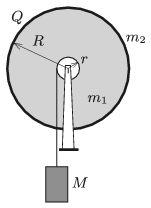(5 pont)

solution (in Hungarian), statistics

P. 5065. A light ray entering into a spherical water drop emerges from the drop perpendicular to its original direction, after two internal reflections, as shown in the figure. What is the angle of incidence? (The refractive index of water is $\displaystyle n=\frac43$.)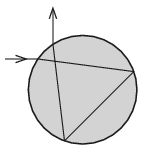(5 pont)

solution (in Hungarian), statistics

P. 5066. In a transparent medium the optical refractive index is changing in the direction of the axis $\displaystyle z$ of the coordinate system. Perpendicular to this, in the direction of the axis $\displaystyle x$ a thin light ray travels, and entering into the medium it is deflected towards the positive region of the axis $\displaystyle z$ along a parabolic path. The refractive index is $\displaystyle n_0$ at $\displaystyle z=0$ and $\displaystyle \sqrt{2}n_0$ at $\displaystyle z=h$. How does the refractive index depend on $\displaystyle z$?

(6 pont)

solution (in Hungarian), statistics